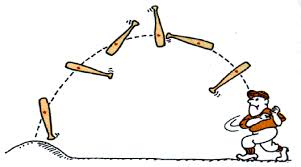# PHYA2 - Mechanics

?
• Created by: Franklin
• Created on: 02-05-14 22:46

## Vectors

A scalar quantity has only magnitude

Examples:

• Temperature
• Distance
• Speed
• Time

A vector quantity has both magnitude and direction

Examples:

• Acceleration
• Velocity
• Momentum
• Force
1 of 11

## Vectors (cont.)

Vectors can be added together to find the resultant vector2 of 11

## Vectors (cont.2)When forces act in a linear fashion like in the image above, the result (resultant force) is just the overall force

3 of 11

## Moments

The moment of a force about any point is the is defined as the force x perpendicular distance from the line of action of the force to the point

Moment of a force = F x d

• Units are newton metres (Nm)
• The greater the distance, the greater the moment

Principle of moments: Sum of the clockwise moments = sum of anticlockwise moments

4 of 11

## Moments 2Taking moments about the pivot: F1 x d1 =  (F2 x d2) +  (F3 x d3)

5 of 11

## Couples

Couples: a pair of equal and opposite forces acting on a body but along the same line. Moment of a couple = torqueMoment of a couple (torque) = F x S

6 of 11

## Centre of Mass

The centre of mass of a body the point through which a single force on the body has no turning effect7 of 11

## Stability

• If a body is in stable equilibrium is displaced then released, it returns to its equilibrium position
• When the body is in equilibrium the reaction of the support and the weight are equal and opposite
• The weight returns to the postion in which it is in the line of action of the support
• In unstable equilibrium the object will not return back to its position of equilibrium

Toppling

• Objects with a high centre of mass are not very stable
• For toppling to occur: Fd >Wb/2
• F = force applied, d = perp dist. from line of action to pivot, W = weight of object, b = width of base
• If the line of action of the weight goes past the pivot the object will topple
8 of 11

## Tilting DiagramIf Fd> Wb/2 toppling will occur (b = width of base)

9 of 11

## Equilibrium Rules

Triangle of forces: Vector sum = 010 of 11

## Projectile Motion

When considering projectile motion, air resistance is usually ingnored. Therefore horizontal velocity is unaffected throughout flight; it is only vertical velocity the changes due to gravity.11 of 11